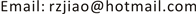﻿ Z[x]的素理想与Krull维数 Prime Ideals and Krull Dimension of Z[x]

Vol.06 No.08(2017), Article ID:22767,4 pages
10.12677/AAM.2017.68113

Prime Ideals and Krull Dimension of $ℤ\left[x\right]$

Rongzheng Jiao

School of Mathematics Science, Yangzhou University, Yangzhou JiangsuReceived: Nov. 4th, 2017; accepted: Nov. 17th, 2017; published: Nov. 23rd, 2017ABSTRACT

Using elementary method, we get all the prime ideals of integral domain $ℤ\left[x\right]$ , which give an explicit proof of a result in Mumford’s red book. We get the Krull dimension 2 of $ℤ\left[x\right]$ by direct computation as a by-product.

Keywords:Integral Domain, Prime Ideal, Maximal Ideal, Krull Dimension, Euclid Domain$ℤ\left[x\right]$ 的素理想与Krull维数1. 引言

1) $p$ 不是 ${a}_{n}$ 的因子；2) $p$${a}_{n-1},{a}_{n-2},\cdots ,{a}_{1},{a}_{0}$ 的因子；3) ${p}^{2}$ 不是 ${a}_{0}$ 的因子。

$f\left(x\right)$$ℤ\left[x\right]$ 中的不可约多项式。

A. Cohn也有一个经典判别准则：

$f\left(x\right)={a}_{m}{x}^{m}+{a}_{m-1}{x}^{m-1}+\cdots +{a}_{0}$$ℤ\left[x\right]$ 上不可约。(文献  )

1981年Brillhart, Filaseta, Odlyzko  将此结果推广成任意在 $b$ -进制。

$f\left(x\right)={a}_{m}{x}^{m}+{a}_{m-1}{x}^{m-1}+\cdots +{a}_{0}$$ℤ\left[x\right]$ 上不可约。

M. R. Murty  还进一步推广至有限域上的多项式。

2000年M. Cavachi  中证明了

$f\left(x\right)+pg\left(x\right)$$ℤ\left[x\right]$ 上除了有限个素数 $p$ 外不可约。

2006年A. I. Bonciocat 与N. C. Bonciocat  给出：

${p}^{m}>|{a}_{n}{a}_{n-2}|{p}^{3e}+{\sum }_{i=3}^{n}|{a}_{n}^{i-1}{a}_{n-i}|{p}^{ie}$ ，且 $m$$e$ 的奇偶性不同。则 $f\left(x\right)$$ℤ\left[x\right]$ 上不可约。

2013年J. Harrington与L. Jones  给出了

2. Z[x]中素理想分类

1) $ℤ\subset ℤ\left[x\right]$$ℤ$ 中的素数 $p$ 是其中的素元，当然是 中不可约的。看成 $ℤ\left[x\right]$ 中的元素时，在 $ℤ\left[x\right]$ 中也是不可约的。所以其生成的理想看成 $ℤ\left[x\right]$ 的理想时也是 $ℤ\left[x\right]$ 中的素理想。所以{(p)│p是素数}是 $ℤ\left[x\right]$ 中的素理想。

2) 如果 $g\left(x\right)$$ℤ\left[x\right]$ 中的不可约多项式，则由它生成的主理想 $\left(g\left(x\right)\right)$$ℤ\left[x\right]$ 中的素理想。

3) $ℤ\left[x\right]$ 中的极大理想形如 $\mathfrak{M}=\left(q,f\left(x\right)\right)$ ，其中了 $q$ 是素数， $f\left(x\right)$$ℤ\left[x\right]$ 中的一个不可多项式，且 $f\left(x\right)$ 模q是 ${ℤ}_{q}\left[x\right]$ 中的不可约多项式。

3. Z[x]的Krull维数

Prime Ideals and Krull Dimension of Z[x][J]. 应用数学进展, 2017, 06(08): 942-945. http://dx.doi.org/10.12677/AAM.2017.68113

1. 1. 波利亚•舍贵. 分析中的问题与定理, 第二卷[M]. 张奠宙, 等, 译. 上海: 上海科技出版社, 1985.

2. 2. Brillhart, J., Filaseta, M. and Odlyzko, A. (1981) On Irreducibility Theorem of A. Cohn. Canadian Journal of Mathematics, 33, 1055-1059.
https://doi.org/10.4153/CJM-1981-080-0

3. 3. Murty, M.R. (2002) Prime Numbers and Irreducible Polynomials. The American Mathematical Monthly, 109, 452-458.
https://doi.org/10.2307/2695645

4. 4. Cavachi, M. (2000) On a Special Case of Hilbert’s Irreduciblility Theorem. Journal of Number Theory, 82, 96-99.

5. 5. Bonciocat, A.I. and Bonciocat, N.C. (2006) Some Classes of Irreducible Polynomials. Acta Arithmetica, 123, 349-360.
https://doi.org/10.4064/aa123-4-4

6. 6. Harrington, J. and Jones, L. (2013) A Class of Irreducible Polynomials. Colloquium Mathematicum, 132, 113-119.
https://doi.org/10.4064/cm132-1-9

7. 7. Munford, D. (1999) The Red Book of Varieties and Schemes. 2nd Edition, Springer, Berlin, 74-75.
https://doi.org/10.1007/b62130

8. 8. Eisenbud, D. (2004) Commutative Algebra with a View toward Algebraic Geometry. Springer, Berlin.